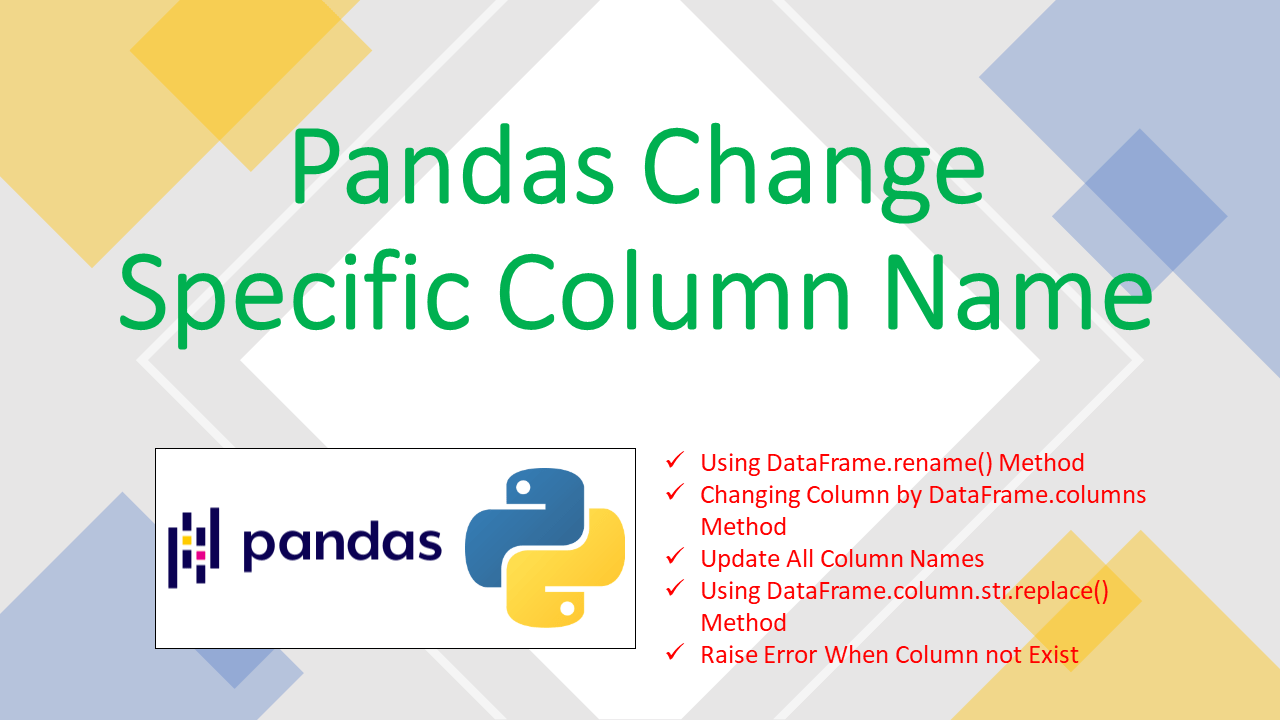# How to Change Column Name in pandas

• Post author:
• Post category:Pandas

You can change the column name of Pandas DataFrame by using the DataFrame.rename() method and the DataFrame.columns() method. In this article, I will explain how to change the given column name of Pandas DataFrame with examples.

• Use the pandas DataFrame.rename() function to modify specific column names.
• Set the DataFrame `columns` attribute to your new list of column names.

## 1. Quick Examples of Change Column Name

If, you are in hurry below are some quick examples to change specific column names on DataFrame.

``````
# Below are some quick examples.

# Syntax to change column name using rename() function.
df.rename(columns={"OldName":"NewName"})

# Using rename() function.
df.rename(columns = {'Fee': 'Fees'}, inplace = True)

# Renaming Multiple columns.
df.rename({'Courses': 'Course_ Name','Fee': 'CourseFee', 'Duration': 'CourseDuration'},
axis = "columns", inplace = True)

# Changing Column Attribute.
df.columns.values = 'Course'

# Errors parameter to 'raise' when column not present.
df2 = df.rename(columns={'Courses': 'EmpCourses'},errors='raise')
``````

Now, let’s create a Pandas DataFrame with a few rows and columns and execute some examples and validate results. Our DataFrame contains column names `Courses`,` Fee` and `Discount`.

``````
# Create a Pandas DataFrame.
import pandas as pd
import numpy as np
technologies= {
'Courses':["Spark","PySpark","Spark","Python","PySpark"],
'Fee' :[22000,25000,23000,24000,26000],
'Duration':['30days','50days','30days','35days','60days']
}
df = pd.DataFrame(technologies)
print(df)
``````

Yields below output.

``````
# Output:
Courses    Fee Duration
0    Spark  22000   30days
1  PySpark  25000   50days
2    Spark  23000   30days
3   Python  24000   35days
4  PySpark  26000   60days
``````

## 2. Using DataFrame.rename() Method

The pandas DataFrame.rename() function is a quite versatile function used not only to rename column names but also row indices. The good thing about this function is that you can rename specific columns. The syntax to change column names using the rename function is-

``````
# Syntax to change column name using rename() function.
df.rename(columns={"OldName":"NewName"})
``````

The `rename()` function returns a new DataFrame with renamed axis labels (i.e. the renamed columns or rows depending on usage). To modify the DataFrame in-place set the argument `inplace` to `True`.

``````
# Using rename() function.
df.rename(columns = {'Fee': 'Fees'}, inplace = True)
print(df)
``````

Yields below output.

``````
# Output:
Courses   Fees Duration
0    Spark  22000   30days
1  PySpark  25000   50days
2    Spark  23000   30days
3   Python  24000   35days
4  PySpark  26000   60days
``````

## 3. Changing Column by DataFrame.columns Method

You can also update the DataFrame column by setting its `columns` attribute to your new list of columns. Access the index to change the specified column name.

``````
# Changing Column Attribute.
df.columns.values = 'Course'
print(df)
``````

Yields below output.

``````
# Output:
Course    Fee Duration
0    Spark  22000   30days
1  PySpark  25000   50days
2    Spark  23000   30days
3   Python  24000   35days
4  PySpark  26000   60days
``````

## 4. Update All Column Names

In this the updated column names as a list to rename the columns. The length of the list we provide should be the same as the number of columns in the DataFrame. Otherwise, an error occurs. For example-

``````
# Using new list of column names
df.columns = technologies
print(df)
``````

Yields below output.

``````
# Output:
Courses    Fee Duration
0    Spark  22000   30days
1  PySpark  25000   50days
2    Spark  23000   30days
3   Python  24000   35days
4  PySpark  26000   60days
``````

## 5. Using DataFrame.column.str.replace() Method

If the number of columns in the Pandas DataFrame is huge, say nearly 100, and we want to replace the space in all the column names (if it exists) by an underscore and it is not easy to provide a list or dictionary to rename all the columns. Then we use the following method-

``````
# Using DataFrame.column.str.replace() Method.
df2 = df.columns.str.replace(' ', '_')
print(df2)
``````

Yields below output.

``````
# Output:
Index(['Courses', 'Fee', 'Duration'], dtype='object')
``````

## 6. Raise Error When Column not Exist

When the column you wanted to change doesn’t exist, no error is raised by default. use raise parameter to raise an error.

``````
# Errors parameter to 'raise'.
df2 = df.rename(columns={'Courses': 'EmpCourses'},errors='raise')
print(df2)
``````

Yields output same as above.

## 7. Complete Examples of Change Column Name of Pandas

``````
# Below are complete examples.
# Create a Pandas DataFrame.
import pandas as pd
import numpy as np
technologies= {
'Courses':["Spark","PySpark","Spark","Python","PySpark"],
'Fee' :[22000,25000,23000,24000,26000],
'Duration':['30days','50days','30days','35days','60days']
}
df = pd.DataFrame(technologies)
print(df)

# Syntax to change column name using rename() function.
df.rename(columns={"OldName":"NewName"})
print(df)

# Using rename() function.
df.rename(columns = {'Fee': 'Fees'}, inplace = True)
print(df)

# Renaming Multiple columns.
df.rename({'Courses': 'Course_ Name','Fee': 'CourseFee', 'Duration': 'CourseDuration'},
axis = "columns", inplace = True)
print(df)

# Change column name using rename() and lambda function.
df2 = df.rename(columns = lambda x: x+':')
print(df2)

# Changing Column Attribute.
df.columns.values = 'Course'
print(df)

# Using new list of column names
df.columns = technologies
print(df)

# Using DataFrame.column.str.replace() Method.
df2 = df.columns.str.replace(' ', '_')
print(df2)

# NO Error is raised.
df2 = df.rename(columns={'Courses': 'EmpCourses'})
print(df2)

# Errors parameter to 'raise'.
df2 = df.rename(columns={'Courses': 'EmpCourses'},errors='raise')
print(df2)
``````

## Conclusion

In this article, You have learned about how to change the specific column name of Pandas DataFrame by using `DataFrame.rename()` method and attributes `DataFrame.column` with some examples.

## References